# Orthogonal

## The Dual Pythagorean Theorem

Much of the physics in the universe of Orthogonal can be understood as arising from fairly simple ideas in geometry. Although the physical consequences are sometimes very different from the consequences in our own universe, the geometry itself is still the same. This page describes one very simple example, which nonetheless underlies one of the most important scientific discoveries in the novel. It’s also a result well worth knowing for its applications in our own world.Let’s start with a geometrical problem that almost everyone knows how to solve at a glance. Suppose we’re told that a car travels along a straight road that is aligned neither exactly north-south nor exactly east-west. The car ends up 3 km to the north of its starting point, but also 4 km to the east of where it began. How far did the car travel?

Pythagoras’s Theorem tells us that the square of the hypotenuse of a right triangle equals the sum of the squares of the other two sides. This gives us the answer straight away: the squares of 3 and 4 are 9 and 16 respectively, and their sum is 25, which is the square of 5. So the total distance the car travels is 5 km.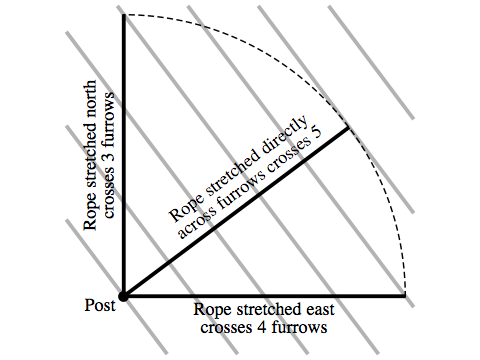Next, consider a field that has been ploughed with evenly spaced, parallel furrows that do not run exactly north-south nor exactly east-west. Starting from a post driven into the ground, if I stretch out a length of rope in a northerly direction I find that it crosses exactly 3 complete furrows. If I stretch the same length of rope in an easterly direction, I find that it crosses 4 complete furrows. The question is this: if I chose a direction for the rope such that it went straight across the furrows, perpendicularly, how many would it cross?

If you were tempted to reply “five” by analogy with the previous problem, then your answer is absolutely right — but if you believed this was just another straightforward application of Pythagoras’s Theorem, then you should look carefully at the diagram. There is no right triangle here with side lengths of 3, 4 and 5 equal units!

Yet the answer is not a misleading coincidence; if we replaced the count of furrows crossed north and east with any values n and e, then the count, f, of furrows crossed directly by the same length of rope would satisfy the equation f 2 = e2 + n2. But if this isn’t merely an example of Pythagoras’s Theorem, where exactly does that relationship come from?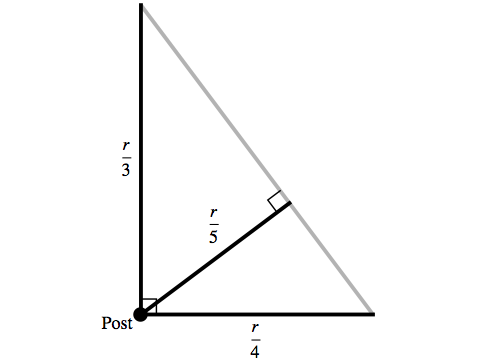If we look at a right triangle whose corner is the post, whose hypotenuse is formed by the first furrow past the post, and whose sides run directly north and east, the lengths of those sides will be r/3 and r/4 respectively, where r is the length of the rope.

A line that joins the right angle of the triangle to the hypotenuse, meeting the hypotenuse at a right angle, will have a length of r/5.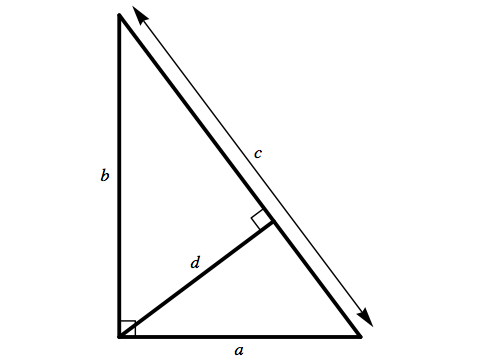It’s worth switching to the general case now, where we have a right triangle with side lengths a and b, hypotenuse c, and a perpendicular distance from the right angle to the hypotenuse of d.

Pythagoras’s Theorem gives us the relationship between a, b and c: c2 = a2 + b2. But what is the relationship between a, b and d?

While a, b and c are all side lengths of the triangle, a, b and d aren’t really the odd triple they might look like at first. What they have in common is that they are all altitudes of the triangle: perpendicular distances from sides of the triangle to the vertex opposite that side. This gives us a clue to an easy way to see how they’re related. The area of a triangle is equal to half its base times its height, or altitude, relative to that base: in other words, half the length of any side, multiplied by the perpendicular distance from that side to the opposite vertex.

This fact gives us two different expressions for the area of the triangle, which must agree:

 (1/2) a b = (1/2) c d

If we double both sides, then square them, we get:

 a2 b2 = c2 d2 a2 b2 = (a2 + b2) d2 1 / d2 = (a2 + b2) / (a2 b2)

where in going from the first line above to the second we’ve used Pythagoras’s Theorem, and in going from the second to the third we’ve divided both sides of the equation by a2 b2 d2. If we divide through by the denominator in the right-hand side of the last line above, we get:

 Dual Pythagorean Theorem 1 / d2 = 1 / a2 + 1 / b2

This relationship applies to any right-angled triangle whose altitudes measured from the two perpendicular sides are a and b, and whose altitude measured from the hypotenuse is d.

Now we can see exactly why our counts of furrows crossed by the same length of rope in the north and east directions, n and e, and the count crossed directly, f, obey the relationship f 2 = e2 + n2. The corresponding distances for the triangle at the post become:

 a = r / e b = r / n d = r / f

and then the Dual Pythagorean Theorem gives us:

 1 / d2 = 1 / a2 + 1 / b2 f 2 / r2 = e2 / r2 + n2 / r2 f 2 = e2 + n2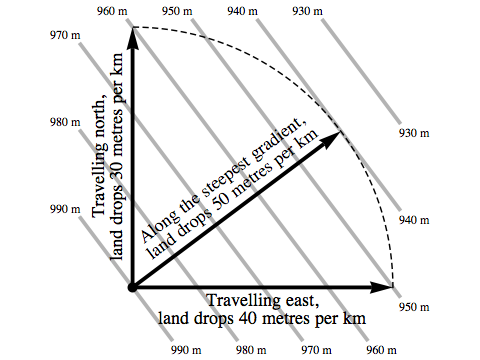Why is this result so important in physics, both in the universe of Orthogonal and in our own universe? What it is telling us is that the sum-of-squares formula of Pythagoras’s Theorem is obeyed not only by quantities like length, but also by “dual” quantities that count how many things happen per some unit of length, or how much something changes per unit of length.

We could replace our furrows in a ploughed field with the lines of constant elevation on a topographic contour map. The Dual Pythagorean Theorem then tells us, for example, that if we’re standing on terrain that drops 30 metres for each kilometre we travel north, and drops 40 metres for each kilometre we travel east, then (at least if the terrain is near enough to a tilted plane, on the scale we’re looking at), its steepest slope, moving across the contours of elevation directly, would have a gradient of 50 metres per kilometre.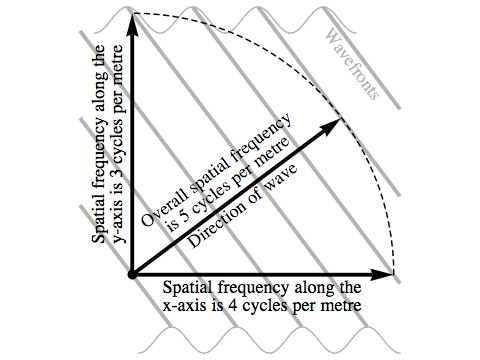The most important application of this result, though, is probably in the geometry of waves. If we replace our furrows with wavefronts (such as the crests of an ocean wave, or the peaks of an electromagnetic wave), then the counts of the number of wavefronts that occur in a given distance are known as spatial frequencies (as opposed to ordinary time frequencies that count the number of oscillations that occur in a given time).

The Dual Pythagorean Theorem tells us that we can find the overall spatial frequency of a wave — the number of wavefronts per unit distance, measured in the direction the wave is travelling — by knowing that its square will equal the sum of the squares of the same measurements taken in two or more perpendicular directions.

In our universe, this is a useful thing to know. In the universe of Orthogonal, it revolutionises the understanding of physics.

There is extra material on this topic for readers who don’t mind a slightly higher level of mathematics.Orthogonal / The Dual Pythagorean Theorem / created Wednesday, 6 April 2011
If you link to this page, please use this URL: https://www.gregegan.net/ORTHOGONAL/01/DualPythagorean.html
Copyright © Greg Egan, 2011. All rights reserved.